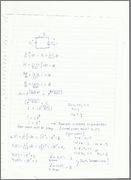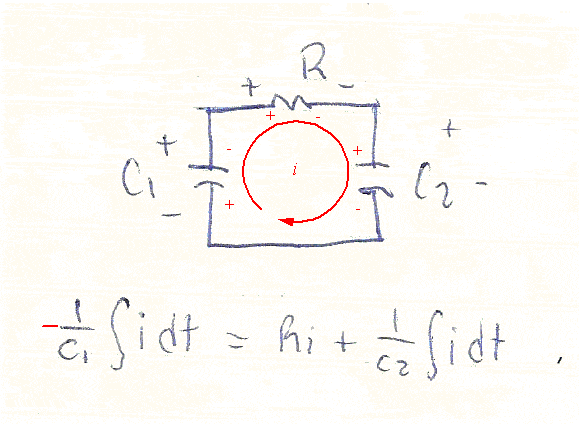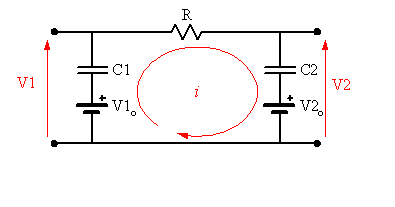# RCC circuit, posible mistake with constants

1f5

## Homework Statement

There's a circuit, with a capacitor C1, Resistor R1, and another capacitor C2.
In t=0 ; C1 is charged with 2 V and C2 with 1 V
The value of resistor is 1 ohm, C1= 1 F C2=0.5 F

## Homework Equations

Kirchoff voltage law.
Capacitor voltage at any given time. (integral form)

## The Attempt at a Solution

I solve the resulting diferential equation, but don't know constant value. I believed that it was -3

One of the things that the problem request if to find a expresion for v1(t), v2(t). I substituted i(t) (diferential equation solution) in the integral form of capacitor voltage formula, well that was easy, but another incise in the problem is to prove that v1(inf)=v2(inf), with the constants from the last integration it is impossible.

Mentor

## Homework Statement

There's a circuit, with a capacitor C1, Resistor R1, and another capacitor C2.
In t=0 ; C1 is charged with 2 V and C2 with 1 V
The value of resistor is 1 ohm, C1= 1 F C2=0.5 F

## Homework Equations

Kirchoff voltage law.
Capacitor voltage at any given time. (integral form)

## The Attempt at a Solution

I solve the resulting diferential equation, but don't know constant value. I believed that it was -3

One of the things that the problem request if to find a expresion for v1(t), v2(t). I substituted i(t) (diferential equation solution) in the integral form of capacitor voltage formula, well that was easy, but another incise in the problem is to prove that v1(inf)=v2(inf), with the constants from the last integration it is impossible.

Is there a circuit diagram to go with this problem? There are several ways to connect three components, and various polarities for the charges on the capacitors.

Last edited:
1f5
RCC pictureLater i discovered that I have a mistake with voltage law, then i resolve the DE and had Ce^(-t/r)
After that i make a sim, and see that the current in t=0 is -1A and tends to zero when t goes to infinity. So the function must be -e^(-t).
(r1=equals 1).

I saw voltages too, but i can't figure from which function they come.

Last edited:
Mentor
The initial current is unlikely to be negative if initially v1 > v2. Also, how do you arrive at a time constant of 1s? By inspection we can see that the current loop has C1 and C2 in series with a resistance R. What's the effective capacitance for C1 and C2 in series? The time constant should then resolve to RCeff when you solve your differential equation.

1f5
Yes, you were right.Mentor
Yes, you were right.Don't let the initial potentials on the capacitors fool you into making wrong choices regarding potential drops due to current flow through them. Whichever plate of a capacitor the current flows INTO with be the + plate as far as the potential drop for the differential equation is concerned.If you want to make it more obvious, note that you can model a pre-charged capacitor as an uncharged capacitor in series with a voltage supply: